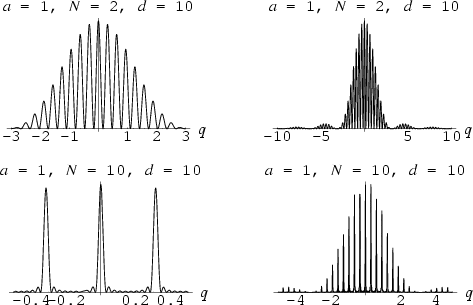# Envelope functions

Hi,

can anyone explain to me what the purpose of finding the envelope function is? I understand graphically that it is a function that is tangent to another curve or another set of curves, but what purpose does it serve?

If we just had one curve, and we found the envelope function, why would we take the effort to find the equation of the envelope rather than just the function itself?

tia

Simon Bridge
Homework Helper
They help you make sense of a complicated function - such as beats in sound waves.

let's say i am to find the envelope of a sinusoidal wave. would the envelope be the peak of the sinusoid or the bottom? would you not lose some information about your sinusoid in that case?

does all the envelope function do is model a simplified version of the original wave?

Last edited:
Simon Bridge
Homework Helper
That's the situation with beats.

The high-frequency wave produces the tone you hear and the low frequency envelope varies how loud the tone is ... giving it a "waa-waa-waa" effect.

In AM radio - the high frequency part is the carrier wave and the envelope carries the information.

Sometimes the envelope is zero when the contained wave would otherwise have a peak - so, yep, the peak there is suppressed. Usually you have enough information to reconstruct the missing part of the underlying function.

You see this in fraunhoffer diffraction, for eg. The fringe spacing is given by a simple relation but the brightness of the fringes is given by an envelope function. Sometimes the envelope suppresses a fringe and you get a bigger than normal gap.

eg.The graphs are intensity with distance. See the effect of the envelope and notice where sometimes fringes (peaks) get suppressed sometimes.

hmm ok, but i guess my question is really more related to modelling a function with an envelope function...
lets say i found the transfer function of the envelope, would that be close enough to model the original wave such that i could fully describe the original wave?

thanks again for the help

Simon Bridge
Homework Helper
No. Look at the bottom-right diagram in the img [last post]. Imagine modelling that knowing only the envelope. Can't be done.

You can model parts of the behavior of the system - depending on what it is. eg. if you have only the envelope function of an am signal, then you can play it as audio.

Usually, though, you task is to separate the envelope from the function for some purpose.

regarding your last comment, my impression that taking the envelope of the function was for the purpose of simplifying a complicated curve, which therefore, should be similar to the original representation.

it seems that (lets say that the q axis is frequency), that if we only really care about the fundamental frequency up to the 3rd harmonic, it looks like we have a fairly good representation of the original signal (top left and top right diagrams, i am assuming the y-axis has the same scale for all the plots) Please correct me if you think this is an incorrect observation.

however, this argument seems to fall apart when observing the bottom left and right diagrams. so because of this, im not really sure if there some metric we can apply to see if a model is correct without actually knowing both the original and the envelope functions.

Simon Bridge
Homework Helper
You misunderstand. Extracting the envelope helps understand the complicated curve. It is still complicated.

q axis is not frequency - it is space. Those are diffraction patterns.
The parameters are width of the slits, number of slits, and slit separation.
http://scienceworld.wolfram.com/physics/DiffractionGrating.html

You'd have to compare the model with the actual measured distribution - however, if you only had the envelope function, you could test those parts of the model that give rise to it.

You could check the model against the underlying physics - eg. see the diffraction grating page above.